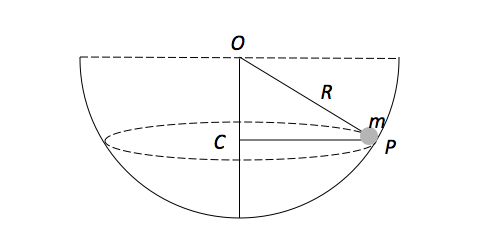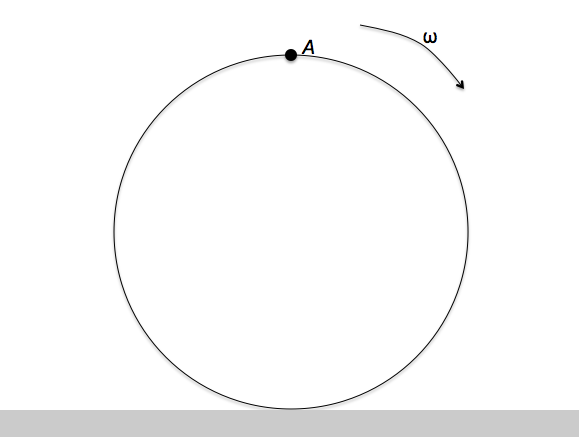Classical Mechanics

# Circular Motion DynamicsA small sphere of mass $m$ is moving on the inner surface of a large hemispherical bowl of radius $R ,$ along a horizontal circle equidistant from the center of the bowl $O.$ As shown in the above diagram, the distance from $O$ to a point $P$ on the circle is $R$ and the distance between $O$ and the center of the circle $C$ is $\lvert\overline{OC}\rvert = \frac{R}{2}.$ What is the force exerted by the sphere on the bowl?

Take gravitational acceleration as $g.$A disc of radius $r = 50 \text{ cm}$ is rotating about its axis with an angular speed of $40 \text{ rad/s}.$ It is gently placed on a perfectly frictionless horizontal surface like in the figure above. Find the linear speed of point $A.$

Old time mills for grinding wheat or corn into flour were often powered by a water wheel. Some water wheels had water falling over paddles, other water wheels dipped their paddles in a moving river, which as it flowed past turned the wheel. One particular mill has a water wheel with radius $3~\mbox{m}$ suspended above a river such that the bottom edge of the paddles are just in contact with and move with the river water. If the river flows at $0.5~\mbox{m/s}$ then what is the period of the spinning water wheel?

A roller coaster works by gravitational energy. The coaster car is pulled up to a high point and then released, rolling downwards on the track through all manners of curves and loops. I have a short roller coaster car that I pull up to the top of a hill of height $H$. The coaster car is released from this height and must go around a perfectly circular vertical loop with a radius of 20 meters (and the bottom of the loop is on the ground). If I don't want the coaster car to fall off the loop at any point, what should be the minimum value of $H$ in meters?

Details and assumptions

• Neglect friction and air resistance.
• Treat the roller coaster car as a point.

What is the maximum speed with which a $1600 \text{ kg}$ car can make a left turn around a curve of radius $49 \text{ m}$ on a level (unbanked) road without sliding?

The static friction constant between the car and the road is $0.1,$ and the gravitational acceleration is $g = 10 \text{ m/s}^2.$

×